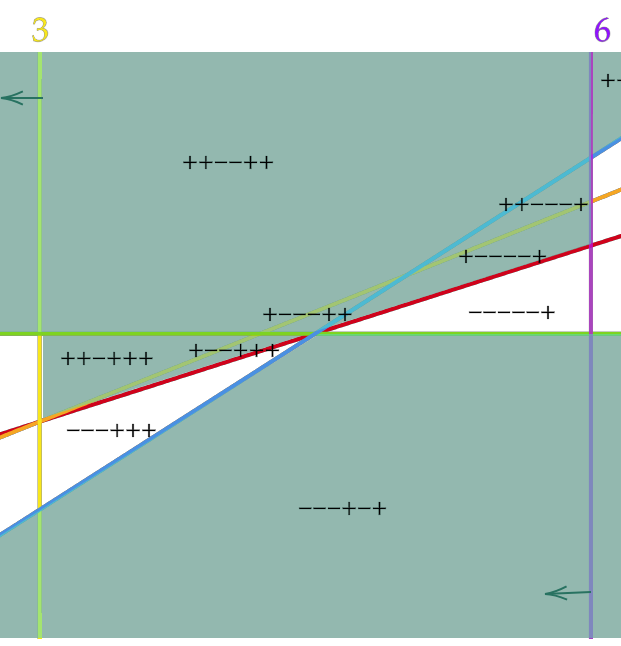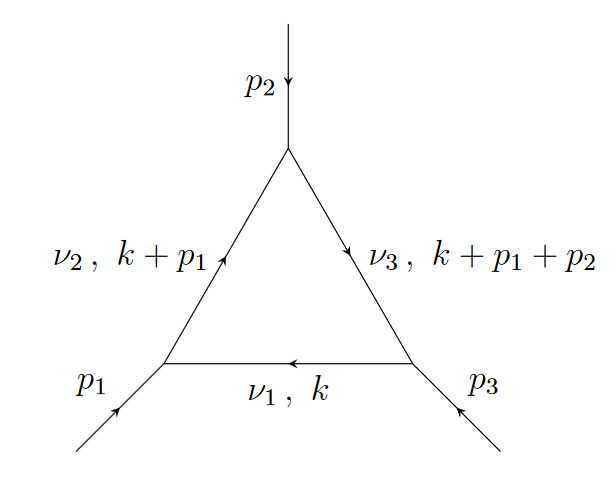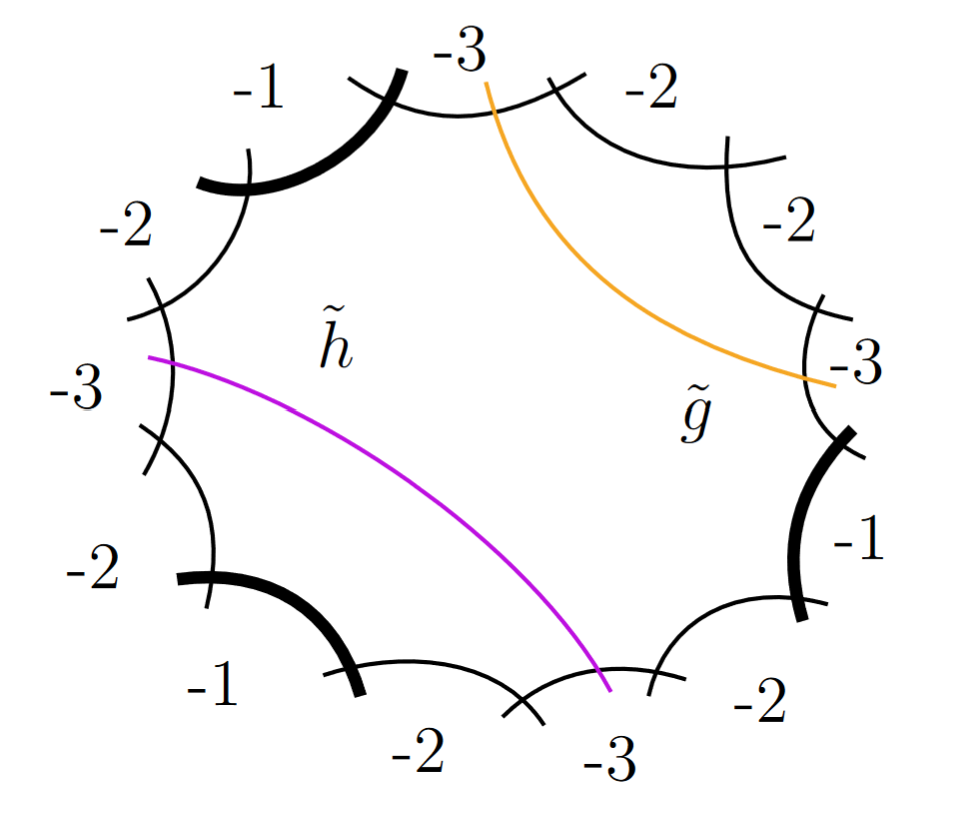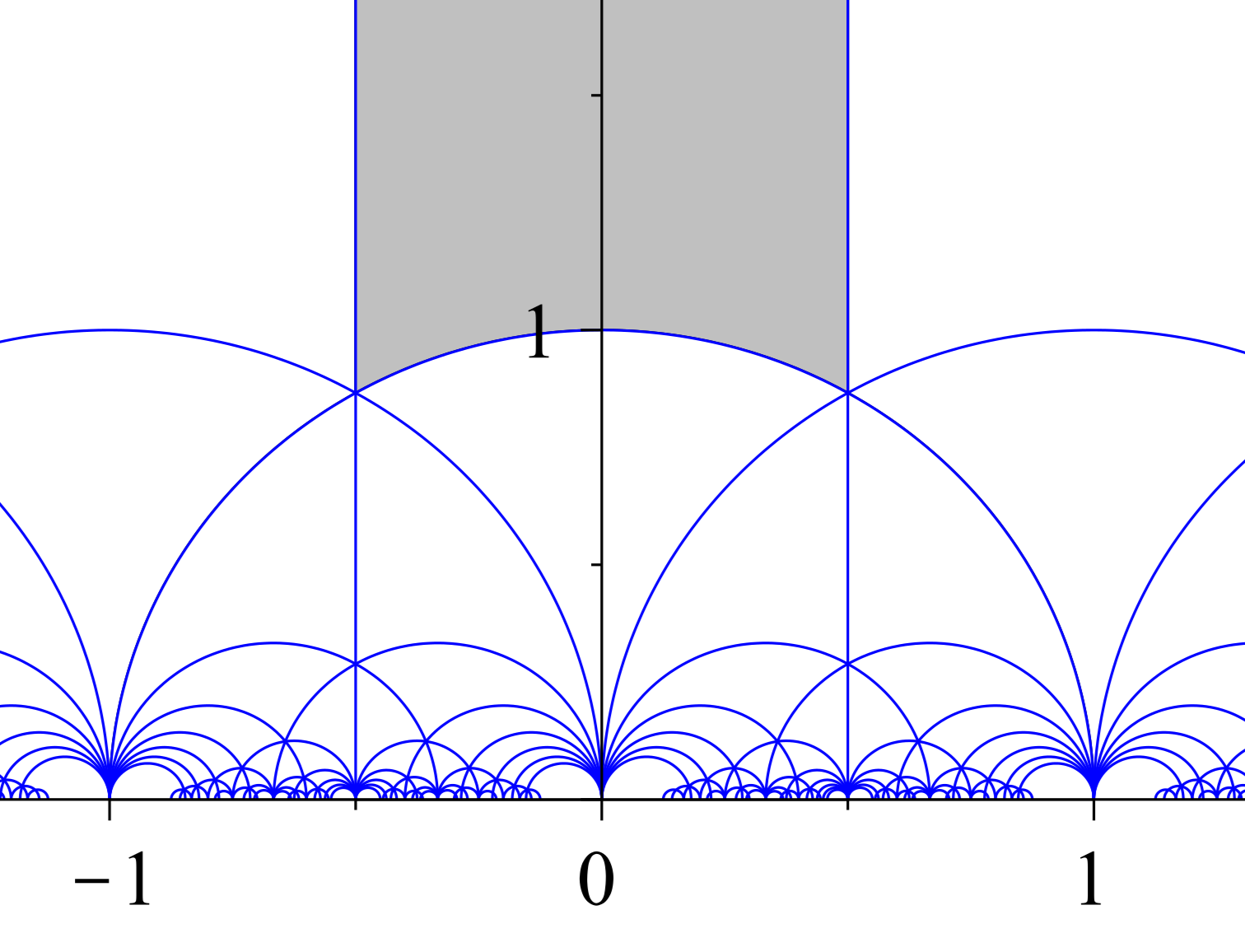# Research

I am broadly interested in combinatorial algebraic geometry.

## Papers:Combinatorics of m=1 Grasstopes (preprint, with Yelena Mandelshtam and Dmitrii Pavlov)

Grasstopes are a generalization of amplituhedra. Building on work of Karp and Williams on the m=1 amplituhedron, we show that m=1 Grasstopes consist of regions of a hyperplane arrangement characterized by a sign condition.D-Module Techniques for Solving Differential Equations in the Context of Feynman Integrals (preprint, with Johannes Henn, Anna-Laura Sattelberger, and Simone Zoia)

We use D-module methods to construct series solutions to linear differential equations appearing in the context of Feynman integrals.In this paper we classify toric elliptic pairs of Picard number two. These elliptic pairs are used to construct examples of surfaces whose pseudo-effective cone is non-polyhedral for a set of primes of positive density, and, assuming the generalized Riemann hypothesis, polyhedral for a set of primes of positive density.Quantum Jacobi forms and sums of tails identities (2022, with Amanda Folsom, Noah Solomon, and Andrew Tawfeek)

We discover new examples of hypergeometric series which are quantum Jacobi forms, i.e. satisfy a modular transformation property up to an analytic error term.